# Thunderstorm

The sound travels 1 km in about 3 seconds. How far is the storm if there is a time interval of 8 seconds between lightning and thunder?

Result

s =  2.667 km

#### Solution:Leave us a comment of example and its solution (i.e. if it is still somewhat unclear...):Be the first to comment!## Next similar examples:

1. Speed of sound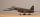The average speed of sound is 330 meters per second. Estimate how long it will hear the church bell 1 km away. Calculate the distance from what would hear sound after 10 seconds.
2. Cyclist 9A cyclist travels at a a speed of 4.25 km per hour. At that rate, how far can he travel in 3.75 hours?
3. Tram lines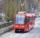Trams of five lines driven at intervals of 5,8,10,12 and 15 minutes. At 12 o'clock come out of the station at the same time. About how many hours again all meet? How many times have earch tram pass for this stop?
4. Round-tripA woman works at a law firm in city A which is about 50 miles from city B. She must go to the law library in city B to get a document. Find how long it takes her to drive​ round-trip if she averages 40 mph.
5. Cars 6At 9:00 am two cars started from the same town and traveled at a rate of 35 miles per hour and the other car traveled at a rate of 40 miles per hour. After how many hours will the cars be 30 miles apart?
6. Bus 14Boatesville is 65.35 kilometers from Stanton. A bus traveling from Stanton is 24.13 kilometers from Boatesville. How far has the bus traveled?
7. Inter city busThe inter city bus leaves Suva at 10.00am and reaches Nadi at 1.00pm covering a distance of 219km. How long did it take to reach the bus Nadi?
8. Average speedMichal went out of the house by car at speed 98 km/h. He came into the goal place for 270 minutes. Determine the distance between the two places.
9. Chocolate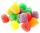I eat 24 chocolate in 10 days. How many chocolate I eat in 15 days at the same pace?
10. Pedestrian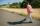Dana started at 10:00 from the point A to point B. These points are distant 12 km. Determine how fast Daniel went when the place B arrived at 11:54. Speed express in km/h.
11. Two cars 2Two cars started from two positions 87 km distant at the same time in opposite directions at speeds 81 km/h and 75 km/h. What was the distance between them after 2 hours 50 minutes of driving.
12. TimeageSeven times of my age is 8 less than the largest two-digit number. How old I am?
13. Pills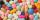If it takes 20 minutes to run a batch of 100 pills how many minutes would it take to run a batch of 50 pills
14. Bed timeTiffany was 5 years old; her week night bedtime grew by ¼ hour each year. If, at age 18, her curfew time is 11pm, what was her bed time when she was 5 years old?
15. How oldThe student who asked how many years he answered: "After 10 years I will be twice as old than as I was four years ago. How old is student?
16. Temperature increaseIf the temperature at 9:am is 50 degrees. What is the temperature at 5:00pm if the temperature increases 4 degrees Fahrenheit each hour?
17. BicycleThe bicycle pedal gear has 36 teeth, the rear gear wheel has 10 teeth. How many times turns rear wheel, when pedal wheel turns 120x?